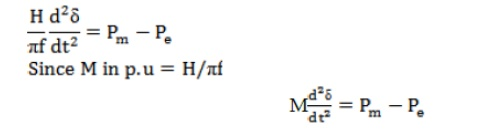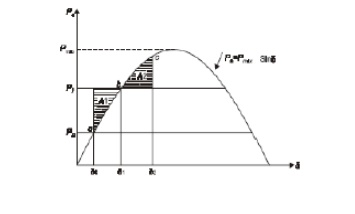Home | | Power System Analysis | Swing equation for a SMIB (Single machine connected to an infinite bus bar) system

# Swing equation for a SMIB (Single machine connected to an infinite bus bar) system

The swing curve is the plot or graph between the power angle δ and time t. From the nature of variations of δ the stability of a system for any disturbance can be determined.

Swing equation for a SMIB (Single machine connected to an infinite bus bar) system.Where H = inertia constant in MW/MVA

f = frequency in Hz

M = inertia constant in p.u

## Swing curve

The swing curve is the plot or graph between the power angle δ and time t. From the nature of variations of δ the stability of a system for any disturbance can be determined.3 machine system having ratings G1, G2 and G3 and inertia constants M1, M2 and M3.What is the inertia constants M and H of the equivalent system.Where G1, G2, G3 – MVA rating of machines 1, 2, and 3

Gb = Base MVA or system MVA

## Assumptions made in stability studies.

(i). Machines represents by classical model

(ii). The losses in the system are neglected (all resistance are neglected)

(iii). The voltage behind transient reactance is assumed to remain constant.

(iv). Controllers are not considered ( Shunt and series capacitor )

(v). Effect of damper winding is neglected.

## Equal Area Criterion

The equal area criterion for stability states that the system is stable if the area under P – δ curve reduces to zero at some value of δ.

This is possible if the positive (accelerating) area under P – δ curve is equal to the negative (decelerating) area under P – δ curve for a finite change in δ. hence stability criterion is called equal area criterion.Study Material, Lecturing Notes, Assignment, Reference, Wiki description explanation, brief detail
Power System Analysis : Stability Analysis : Swing equation for a SMIB (Single machine connected to an infinite bus bar) system |